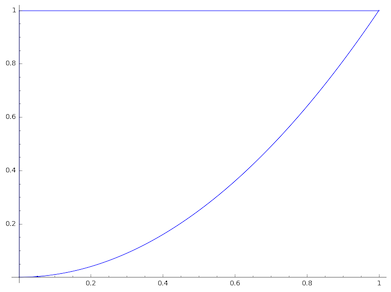# Closed integral x^2y^2 dx + xy dy, C consists of arc of the parabola y = x^2 from (0, 0) to (1,...

## Question:

Consider the integral {eq}\displaystyle \oint_C x^2y^2\ dx + xy\ dy {/eq}, where {eq}C {/eq} consists of the arc of the parabola {eq}y = x^2 {/eq} from {eq}(0,\ 0) {/eq} to {eq}(1,\ 1) {/eq} followed by the line segment from {eq}(1,\ 1) {/eq} to {eq}(0,\ 1) {/eq} and the line segment from {eq}(0,\ 1) {/eq} to {eq}(0,\ 0) {/eq}.

Evaluate the line integral by two methods:

(a) Directly.

(b) By applying Green's Theorem.

## Green's Theorem:

Let {eq}C {/eq} be an oriented simple closed curve which is the oriented boundary of the region {eq}R {/eq}. Suppose that {eq}\vec{F}=\left<P,Q\right> {/eq} is a vector field whose domain contains the entire region {eq}R {/eq}. Then Green's theorem is the following integral equality:

{eq}\displaystyle \oint_C \vec{F} \cdot ds = \iint_R \left(\frac{\partial Q}{\partial x}-\frac{\partial P}{\partial y}\right) \, dA \, . {/eq}

Green's theorem can be thought of as a higher-dimensional version of the fundamental theorem of calculus. Where the fundamental theorem of calculus applies to intervals on the real line, Green's theorem applies to regions in the plane.

## Answer and Explanation:

(a) Let {eq}C_1 {/eq} be the parabolic arc from {eq}(0,0) {/eq} to {eq}(1,1) {/eq}; let {eq}C_2 {/eq} be the line segment from {eq}(1,1) {/eq} to {eq}(0,1) {/eq}; and let {eq}C_3 {/eq} be the line segment from {eq}(0,1) {/eq} to {eq}(0,0) {/eq}. Then

{eq}\displaystyle \oint_C (x^2y^2 \, dx + xy \, dy)=\int_{C_1} (x^2y^2 \, dx + xy \, dy)+\int_{C_2} (x^2y^2 \, dx + xy \, dy)+\int_{C_3} (x^2y^2 \, dx + xy \, dy) \, . {/eq}

We'll evaluate each of these line integrals separately.

First, parameterize the parabolic arc {eq}C_1 {/eq} by the function {eq}r(t)=(t,t^2) {/eq} for {eq}0 \le t \le 1 {/eq}. Then {eq}r'(t)=\left<1,2t\right> {/eq}, and so

{eq}\begin{align*} \int_{C_1} (x^2y^2 \, dx + xy \, dy) &=\int_0^1 \left[t^2(t^2)^2(1)+t(t^2)(2t)\right] \, dt&\text{(by the definition of the line integral)}\\ &=\int_0^1 (t^6+2t^4) \, dt\\ &=\left(\frac{1}{7}t^7+\frac{2}{5}t^5\right|_0^1&\text{(evaluating the integral)}\\ &=\frac{1}{7}+\frac{2}{5}\\ &=\frac{19}{35} \, . \end{align*} {/eq}

Next, parameterize the line segment {eq}C_2 {/eq} by the function {eq}r(t)=(-t,1) {/eq} for {eq}-1 \le t \le 0 {/eq}. Then {eq}r'(t)=\left<-1,0\right> {/eq}, and so:

{eq}\begin{align*} \int_{C_1} (x^2y^2 \, dx + xy \, dy) &=\int_{-1}^0 \left[(-t)^2(1)^2(-1)+(-t)(1)(0)\right] \, dt&\text{(by the definition of the line integral)}\\ &=\int_{-1}^0 -t^2 \, dt\\ &=\left(-\frac{1}{3}t^3\right|_{-1}^0&\text{(evaluating the integral)}\\ &=-\frac{1}{3} \, . \end{align*} {/eq}

Finally, on the line segment {eq}C_3 {/eq}, the coordinate {eq}x {/eq} is always zero and so the line element {eq}x^2y^2 \, dx + xy \, dy=0y^2 \, dx + 0y \, dy {/eq} is always zero. It follows that {eq}\int_{C_3} (x^2y^2\, dx + xy \,dy)=0 {/eq}.

Putting all this together, we have:

{eq}\begin{align*} \oint_C (x^2y^2 \, dx + xy \, dy)&=\int_{C_1} (x^2y^2 \, dx + xy \, dy)+\int_{C_2} (x^2y^2 \, dx + xy \, dy)+\int_{C_3} (x^2y^2 \, dx + xy \, dy) \\ &=\frac{19}{35}-\frac{1}{3}+0\\ &=\frac{22}{105} \, . \end{align*} {/eq}

(b) The curve {eq}C {/eq} is drawn below:As you can see, the region {eq}R {/eq} bounded by {eq}C {/eq} lies above the parabola {eq}y=x^2 {/eq} and below the line {eq}y=1 {/eq} for {eq}0 \le x \le 1 {/eq}. So the Cartesian coordinate bounds on {eq}R {/eq} are {eq}0 \le x \le 1 {/eq} and {eq}x^2 \le y \le 1 {/eq}.

Now, we can evaluate the integral via Green's theorem as follows:

{eq}\begin{align*} \oint_C (x^2y^2 \, dx + xy \, dy)&=\iint_R \left(\frac{\partial}{\partial x}(xy)-\frac{\partial}{\partial y}(x^2y^2)\right) \, dA&\text{(by Green's theorem)}\\ &=\iint_R (y-2x^2y) \, dA&\text{(evaluating the partial derivatives)}\\ &=\int_{x=0}^1 \int_{y=x^2}^1 (y-2x^2 y) \, dy \, dx&\text{(rewriting the area integral as an iterated integral in Cartesian coordinates)}\\ &=\int_{x=0}^1 \left(\frac{y^2}{2}-x^2y^2\right|_{y=x^2}^1 \, dx&\text{(evaluating the }y\text{-integral)}\\ &=\int_{x=0}^1 \left(\frac{1}{2}-x^2-\frac{x^4}{2}+x^6\right) \, dx\\ &=\left(\frac{1}{2}x-\frac{1}{3}x^3-\frac{1}{10}x^5+\frac{1}{7}x^7\right|_0^1&\text{(evaluating the }x\text{-integral)}\\ &=\frac{1}{2}-\frac{1}{3}-\frac{1}{10}+\frac{1}{7}\\ &=\boxed{\frac{22}{105}} \, . \end{align*} {/eq}

Both methods of evaluating the line integral give the result {eq}\dfrac{22}{105} {/eq}, verifying Green's theorem for this integral.